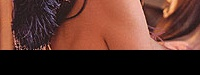# Crop a part of the image with Python, Pillow (trimming)

Posted: 2019-05-14 / Tags: Python, Pillow, Image Processing

In `Image` module of the image processing library Pillow (PIL) of Python, `crop()` for cutting out a partial area of an image is provided.

Here, the following cases will be described with sample code.

• Normal crop
• Specify outside area
• Crop the center of the image
• Crop the largest square from the rectangle

Please refer to the following post for the installation and basic usage of Pillow (PIL).

If you want to create a transparent image by cutting out a shape other than a rectangle (such as a circle), use `putalpha()`.

Import `Image` from `PIL` and open the target image.

```from PIL import Image

im = Image.open('data/src/lena.jpg')
```## Normal crop

Set the cropping area with `box=(left, upper, right, lower)`.

The top left coordinates correspond to `(x, y) = (left, upper)`, and the bottom right coordinates correspond to `(x, y) = (right, lower)`. The area to be cropped is `left <= x <right` and `upper <= y <lower`, and the pixels of `x = right` and`y = lower` are not included.

Be careful not to forget that `box` requires `()`.

```im_crop = im.crop((100, 75, 300, 150))
im_crop.save('data/dst/lena_pillow_crop.jpg', quality=95)
```

If you just want to save the cropped image without using it for other processing, you can write in one line.

```im.crop((100, 75, 300, 150)).save('data/dst/lena_pillow_crop.jpg', quality=95)
```## Specify outside area

If the outside of the image is set in the cropping area, an error does not occur and the image is displayed in black.

```im_crop_outside = im.crop((100, 175, 300, 250))
im_crop_outside.save('data/dst/lena_pillow_crop_outside.jpg', quality=95)
```## Crop the center of the image

If you want to crop the center of the image to any size, it is convenient to define the following function.

```def crop_center(pil_img, crop_width, crop_height):
img_width, img_height = pil_img.size
return pil_img.crop(((img_width - crop_width) // 2,
(img_height - crop_height) // 2,
(img_width + crop_width) // 2,
(img_height + crop_height) // 2))
```
source: imagelib.py

An example of using this function is as follows.

```im_new = crop_center(im, 150, 200)
im_new.save('data/dst/lena_crop_center.jpg', quality=95)
```## Crop the largest square from the rectangle

When creating a thumbnail image, for example, it may be desirable to trim a square as large as possible from the rectangular image.

Defines a function that crop a square of short side length from the center of the rectangular image.

```def crop_max_square(pil_img):
return crop_center(pil_img, min(pil_img.size), min(pil_img.size))
```
source: imagelib.py

An example of using this function is as follows.

```im_new = crop_max_square(im)
im_new.save('data/dst/lena_crop_max_square.jpg', quality=95)
```